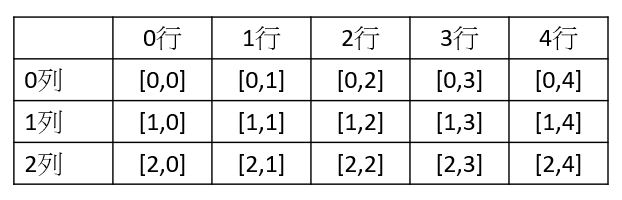# 陣列宣告與建立

int [] score;//宣告
score=new int;//產生10個空間

int[] score=new int;

# 陣列元素使用

for (int i = 0; i < score.Length; i++)
{
Console.WriteLine("{0}", score[i]);
}


# 二維陣列

static void Main(string[] args)
{
int[,] student = new int[10,3];
for (int i = 0; i < student.GetLength(0); i++)
{
for (int j = 0; j < student.GetLength(1); j++)
{
Console.Write("{0} ", student[i, j]);
}
Console.WriteLine();
}
}


int[,] student=new int[10,3]; 最表示宣告10列, 3行的二維陣列. 陣列維度可以最多達到32維.

# 二維陣列的座標# 陣列變數初始化

int[] s;

s=new int
s=100

# 陣列元素初始化

int []s=new int[]{1,2,3,4,5};

int []s={1,2,3,4,5}

int [,] s=new int[,]{{0,0,0,0,0}, {0,0,0,0,0}, {0,0,0,0,0}}

int [,] s=new int[3,5]{{0,0,0,0,0}, {0,0,0,0,0}, {0,0,0,0,0}}, 不過這種方法是多此一舉, 因為系統會自動幫我們算行及列的數目

# 陣列常用的屬性與方法

Length : 屬性, 查陣列總長度

Rank : 屬性, 查陣列維度

GetLength(0) : 方法, 取得某一維度之長度

int[,]a=new int[,]{3,4};
a.Length, 此法在Java為正確的寫法, 但在 C#是錯誤的寫法

# foreach

foreach適用於集合之迴圈

foreach(資料型別 var in 集合物件){}, 請參照如下代碼

static void Main(string[] args)
{
int[] s = { 1, 2, 3, 4, 5 };
foreach (int i in s)
{
Console.WriteLine(i);
}
}


# Array.ForEach()用法

Array.ForEach(陣列, action), 將陣列及action傳入, 此時就會依陣列的元素,  一個一個執行Action的工作.

class Program
{
static void Main(string[] args)
{
int[] r = { 1, 2, 3, 4, 5 };
Action myAction = new Action(Area);
Array.ForEach(r, myAction);
}
static void Area(int r)
{
Console.WriteLine(r*r*Math.PI);
}
}

3.14159265358979
12.5663706143592
28.2743338823081
50.2654824574367
78.5398163397448


# 陣列的排序與搜尋

Array.Sort(Array1, Array2) : 可只有一個Array, 最多二個

Array.Reverse(Array);

# 不等長陣列

static void Main(string[] args)
{
int[][] a = new int[];
a = new int;
a = new int;
a = new int;
for (int i = 0; i < a.Length; i++)
{
for (int j = 0; j < a[i].Length; j++)
{
Console.Write("{0} ", a[i][j]);
}
Console.WriteLine();
}
}


# struct 結構

C#也有跟C語言一樣的struct, 使用方式如下

namespace ConsoleApp1
{
class Program
{
struct Student
{
public string name;
public int chinese;
public int math;
}
static void Main(string[] args)
{
Student s1=new Student();
s1.name = "Thomas";
s1.chinese = 100;
Console.WriteLine("{0},{1}", s1.name, s1.chinese);
}
}
}



struct需要寫於Main()之外, 而且裏面的變數都必需宣告為 public才可以讀取.

namespace ConsoleApp1
{
class Program
{
struct Student
{
public string name;
public int chinese;
public int math;
}
static void Main(string[] args)
{
Student[] bcc = new Student[] {
new Student(){name="Thomas", chinese=100, math=100 },
new Student(){name="Jonh", chinese=70, math=50 },
new Student(){name="Tom", chinese=80, math=100 }
};
foreach(Student s in bcc)
{
Console.WriteLine("{0} : {1},{2}", s.name, s.chinese, s.math);
}
}
}
}

Thomas : 100,100
Jonh : 70,50
Tom : 80,100

# 汽泡排序法(Bubble Sort)

1. 假設共有n個數(0~n-1)
2. 第 i 個數 (0~n-2)必需跟後面比(i+1~n-1), 如果前面的數比較大, 則對調

static void Main(string[] args)
{
int n= 10;
Random r = new Random();
int[] d = new int[n];

//產生10個亂數
for (int i = 0; i < d.Length; i++)
{
d[i] = r.Next(1,100);
}

//列印未排序的陣列
Console.WriteLine("排序前");
foreach(int i in d)
{
Console.Write("{0} ", i);
}
Console.WriteLine();

//排序
for (int i = 0; i < d.Length - 1; i++)
{
for (int j = i + 1; j < d.Length; j++) { if (d[i] > d[j])
{
int temp = d[i];
d[i] = d[j];
d[j] = temp;
}
}
}

//列印已排序的陣列
Console.WriteLine("排序後");
foreach (int i in d)
{
Console.Write("{0} ", i);
}
Console.WriteLine();
}

90 45 32 67 65 8 25 14 78 62

8 14 25 32 45 62 65 67 78 90

Array.Sort(d);